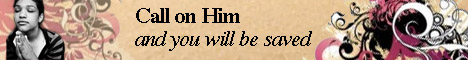Note:  Do not rely on this information. It is very old.

# Equivalent Weights

Equivalent Weights. The atoms of different elements have a varying power of combination with other elements. [Atomic Theory.] Thus one atom of chlorine can combine with one atom of hydrogen, while one atom of oxygen can combine with two, and an atom of carbon with four hydrogen atoms. This power is known as the valency (q.v.) of the atom. Thus chlorine is monovalent, oxygen divalent, nitrogen trivalent, and carbon tetravalent. The atomic weight [Atomic Theory] of an element divided by the valency gives What is known as the equivalent weight - i.e. the weight which can unite with or is equivalent to the part by weight of hydrogen. This can in most cases be found without any regard to theory, thus 35-4 parts chlorine can unite with 1 of hydrogen, and with 39 of potassium. Hence 39 parts of potassium are equivalent to 1 of hydrogen - i.e. the equivalent weight of potassium is 39. This being the statement of an experimental fact, and not depending upon any theoretical basis, the equivalent weights were for a long time used in preference to the atomic Weights, and still are so used by a section of French chemists. Owing, however, to a variable valency many elements possess variable equivalent weights, and by far the majority of chemists have discarded the older system.